maths > wholenumbers

Multiplication: First Principles

what you'll learn...

overview

This page introduces multiplication of whole numbers as -- one number, multiplicand, is repeated the number of times given by the second number, multiplier. The combined result of the repetition is counted or measured as the product of the multiplication.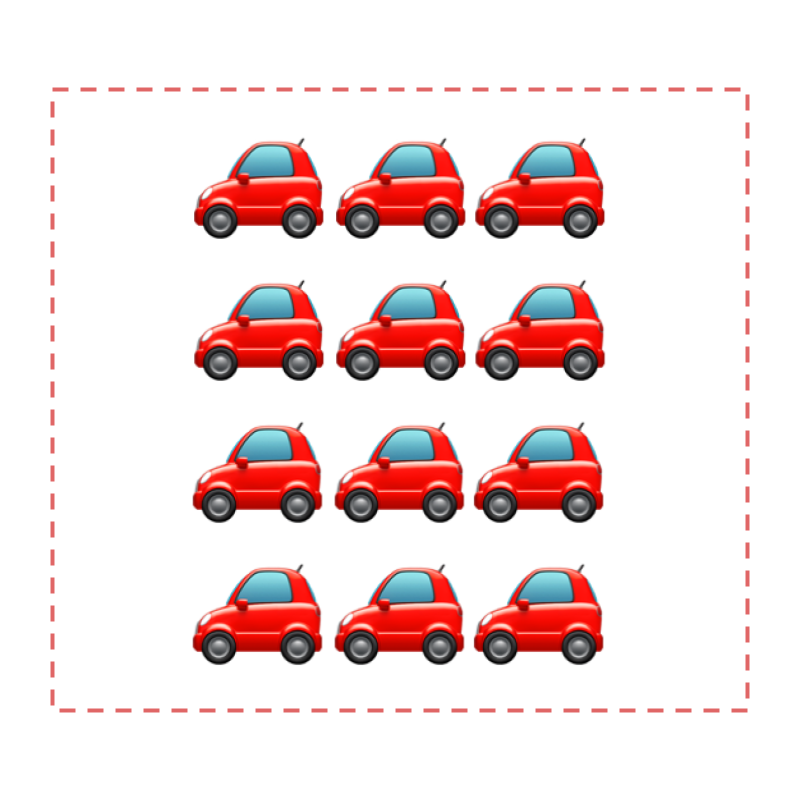Count of $3$$3$ cars is repeated $4$$4$ number of times. The resulting count is $12$$12$.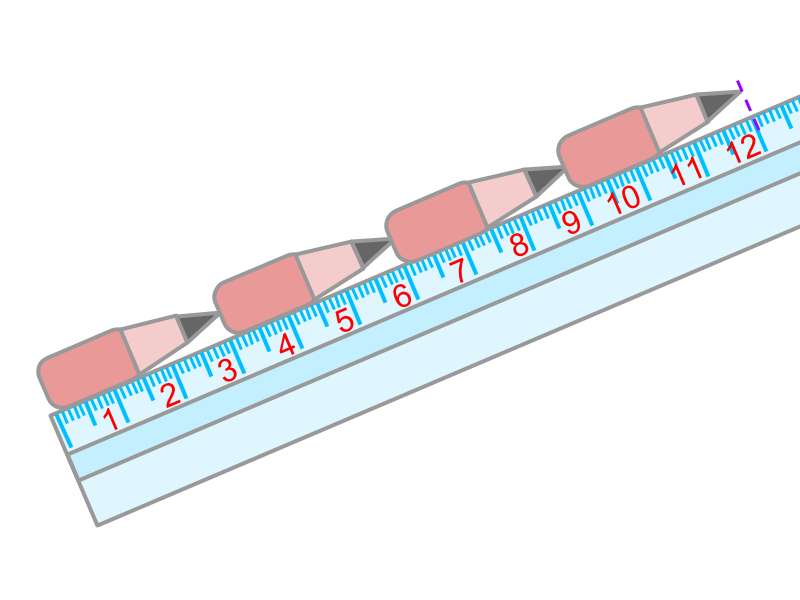Measure of $3$$3$ is repeated $4$$4$ times. The resulting measure is $12$$12$.

The same is abstracted for multiplication in number-line.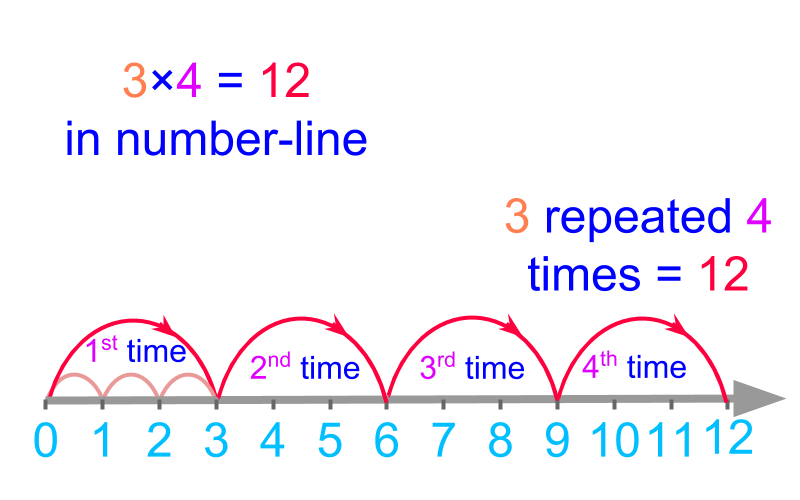The definition of multiplication in first principles form the basis to understanding the simplified procedure for multiplication of large numbers.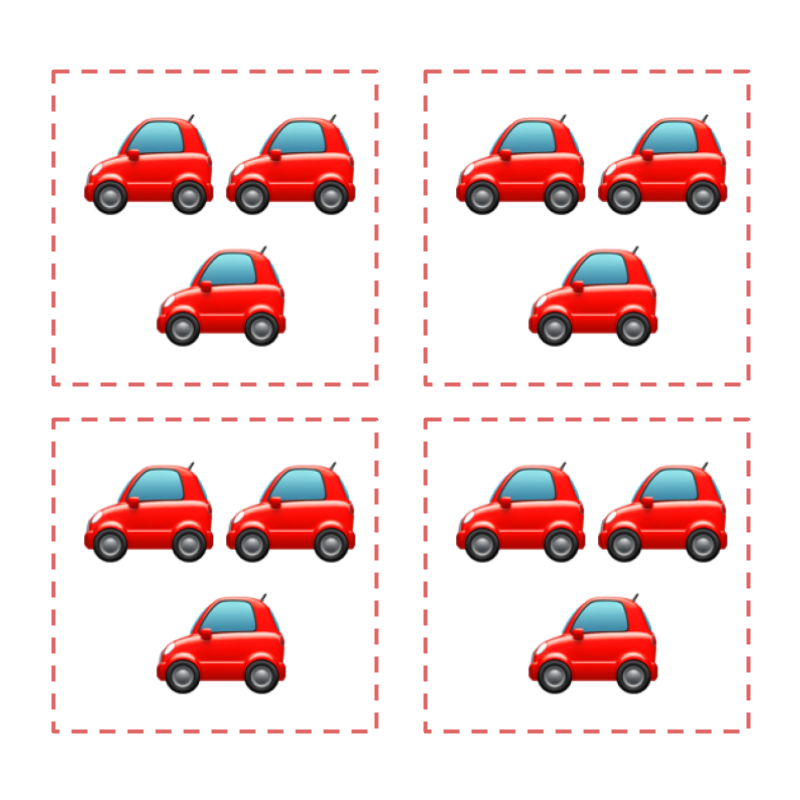$3$$3$ cars were given to $4$$4$ show rooms each.

The total number of cars given are $12$$12$.

The problem is understood to be that of repeated addition.

$3$$3$ cars were given to the first show room, then to the second, then to the third, and then to the fourth. So $3$$3$ was repeatedly added $4$$4$ times to get the total.Considering $3$$3$ cars given to $4$$4$ show rooms each. All the cars are shown in the figure. Counting the number of cars, the result is $12$$12$.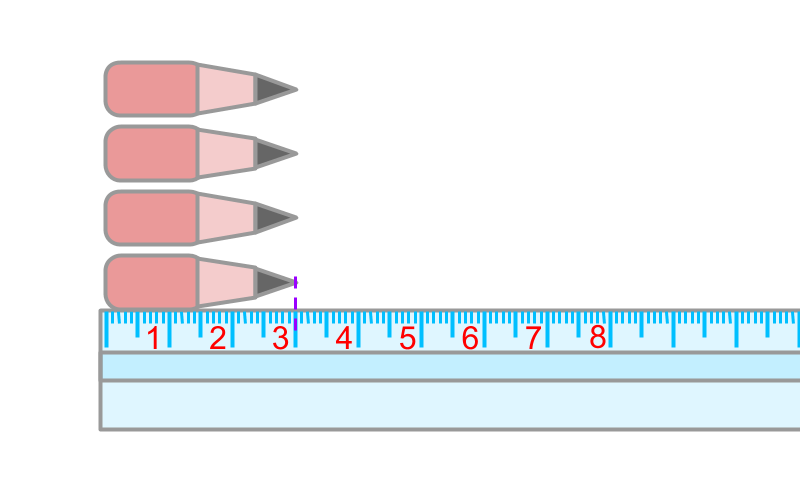There are $4$$4$ pencils, each of $3$$3$ centimeter long.

The combined length of the pencils is $12$$12$ centimeter.

The problem is understood to be that of repeated addition.

Length of one pencil is $3$$3$ centimeters. To get the combined length of $4$$4$ such pencils, the length is repeatedly added $4$$4$ times.Considering $4$$4$ pencils, each of 3 centimeter long. The four pencils are placed side-by-side and the length measurement is taken from $0$$0$. The measure at the end is $12$$12$. The combined length is $12$$12$ centimeters.

The count or measure of a quantity can be repeatedly added or multiplied.

The multiplication results in count or measure of the combined quantity.Multiplication $3×4$$3 \times 4$ in the number-line is given in the figure.

The value $3$$3$ is repeated $4$$4$ times to land in $12$$12$. The result of the multiplication is $12$$12$.

The word "multiplication" means: increase in quantity many times over.

multiplicant, multiplier, product

Multiplication - First Principles : Two numbers are considered, each of which represents a count or measurement. One of the amount is repeated the number of times given by the second amount to form the result representing the collective amount from such repetition.

eg: $12×3=36$$12 \times 3 = 36$

$12$$12$ is the multiplicand

$3$$3$ is the multiplier

$36$$36$ is the product

The word "multiplicand" means: the number or quantity that is repeatedly added in a multiplication.

The word "multiplier" means: the number of times a quantity is repeatedly added.

The word "product" means: the result of multiplication.

summary

Multiplication - First Principles : Two numbers are considered, each of which represents a count or measurement. One of the amount is repeated the number of times given by the second amount to form the result representing the collective amount from such repetition.

eg: $12×3=36$$12 \times 3 = 36$

$12$$12$ is the multiplicand

$3$$3$ is the multiplier

$36$$36$ is the product

Outline